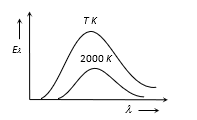Question

# The adjoining diagram shows the spectral energy density distribution ${E}_{\lambda }$ of a black body at two different temperatures. If the areas under the curves are in the ratio 256 : 1, the value of temperature T isModerate
Solution

## $\frac{{A}_{T}}{{A}_{2000}}=\frac{256}{1}$                 (given) Area under ${e}_{\lambda }-\lambda$  curve represents the emissive power of body and emissive power  $\propto {T}^{4}$ (Hence area under   ${e}_{\lambda }-\lambda$ curve)  $\propto {T}^{4}$$⇒\frac{{A}_{T}}{{A}_{2000}}={\left(\frac{T}{2000}\right)}^{4}⇒\frac{256}{1}={\left(\frac{T}{2000}\right)}^{4}⇒T=8000K.$

Get Instant Solutions